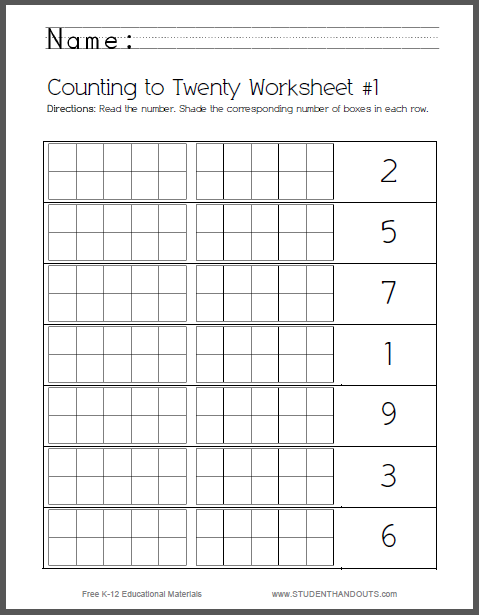Set of 8 Counting-to-Twenty Worksheets for Kids | Student Handouts
 Share the learning joy!

Set of 8 Counting-to-Twenty Worksheets for Kids

The concept here is simple. Students read the number, then shade the corresponding number of boxes. The numbers go up to 20, and the boxes are grouped into ten units, to help students understand numbers in terms of base ten. There are eight of these worksheets in total. Click below to print.There are eight of these base ten counting worksheets to choose from. These are appropriate for teaching kindergarten math counting concepts, and as mathematics review for grade one. The corresponding Common Core State Standards are detailed below, with links to similar free worksheets.

CCSS.MATH.CONTENT.K.NBT.A.1 - Compose and decompose numbers from 11 to 19 into ten ones and some further ones, e.g., by using objects or drawings, and record each composition or decomposition by a drawing or equation (such as 18 = 10 + 8); understand that these numbers are composed of ten ones and one, two, three, four, five, six, seven, eight, or nine ones.

CCSS.MATH.CONTENT.1.NBT.B.2.A - 10 can be thought of as a bundle of ten ones--called a "ten."

CCSS.MATH.CONTENT.1.NBT.B.2.B - The numbers from 11 to 19 are composed of a ten and one, two, three, four, five, six, seven, eight, or nine ones.